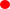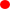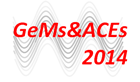﻿ GEMS&ACES ProgramScheduleMonday to Friday:

Morning:

• 9:00 - 11:00: First lecture session
• 11:00 - 11:30: Coffee break
• 11:30 - 13:30: Second lecture session

Afternoon:

• 15:30 - 17:30 PM: Single lecture session

Wednesday :

• 18:00-20:00: Hike to places nearby A Coruña

Monday 9th: Introduction, by J. Brégains

• Matrices and tensor algebra
• Introduction to differential forms
• Maxwell equations in DF formalism

Tuesday 10th: DFs and Geometry, by R. Kotiuga

• Differential forms, variational principles, and topology as a barometer of dimensional issues
• A fictionalized history of the FEM in computational E&M
• Geometric inverse problems

Wednesday 11th: Geometric Methods, by Ch. Geuzaine

• Domain decomposition approaches for high-frequency problems
• Curved meshes

Thursday 12th: Numerical Modelling In Optics, by A. Nicolet

• Principles of transformation optics
• Exotic optical devices: invisibility cloaks and super-lenses
• Perfectly matched layers

Friday 13th: Cell Methods, by B. Auchmann

• Discrete Maxwell equations
• Discrete boundary-value problems / cell method
• Finite elements and cell methods. Applications

GEOMETRIC METHODS, by Ch. Geuzaine

Description

The lecture will touch upon two recent developments for the numerical simulation of electromagnetic problems using finite element methods. In the first part, I will introduce domain decomposition techniques to tackle the open problem of solving large scale high-frequency harmonic wave problems. In the second part I will detail how high-order finite element meshes can be generated on complex domains, in order to minimize the error linked with the approximation of the geometry. Hands-on computer experiments will be proposed for both parts, using Gmsh (http://gmsh.info) and GetDP (http://getdp.info).

References

1. A. Vion, C. Geuzaine, Double Sweep Preconditioner For Optimized Schwarz Methods Applied To The Helmholtz Problem, Journal Of Computational Physics, In Press, 2014.
2. Y. Boubendir, X. Antoine, C, Geuzaine, A Quasi-Optimal Non-Overlapping Domain Decomposition Algorithm For The Helmholtz Equation, Journal Of Computational Physics 231 (2), 262-280, 2012.
3. A. Johnen, J.-F. Remacle, C. Geuzaine, Geometrical Validity Of Curvilinear Finite Elements, Journal Of Computational Physics 233, 359-372, 2013.
4. T. Toulorge, C. Geuzaine, J.-F. Remacle, J. Lambrechts, Robust Untangling Of Curvilinear Meshes, Journal Of Computational Physics 254, 8-26, 2013.

DIFFERENTIAL FORMS AND GEOMETRY, by R. Kotiuga

Description

1. An Informal Introduction by means of Differential Calculus. 2 Differential Forms and Variational Principles. 2.1 Simple Variational Principles. 2.1.1 The basic variational problems. 2.1.2 Direct varaitional methods and the FEM. 2.1.3 Symbols of differential operators and a general form of the multivariable E-L equation. 2.2 Differential forms revisited. 2.2.1 Cohomology, homology and de Rham's theorems. 2.2.2 Duality. 2.2.3 Simplicial complexes and Whitney forms. 2.3 The deRham Complex meets the Riemannian Metric. 2.3.1 Differential Forms and Hodge theory. 2.3.2 Enter the covariant derivative. 2.3.3 The eigenvalue problem revisited. 2.3.4 Square roots of differential operators. 3 A fictionalized history of FEM in CEM with future indications. 3.1 Hodge theory + Whitney forms + FEM = Discrete Hodge Theory. 3.2 Looking forward with spectral geometry. 4 Differential Forms and Electromagnetics in 3+1 = 4 Dimensions. 5 Topology as a Barometer of Dimensional Issues

5.1 1; 2; n; 3; 4; ::: 5.2 From homotopy and homology to Algebraic Topology. 5.3 Revisiting the making cuts in 3-d from an algorithmic perspective. 6 Geometric Inverse Problems. 6.1 Can you hear the shape of... a drum?... a graph? 6.2 Electrical impedance tomography and invese scattering. 6.3 Force-free magnetic fields.

References

1.  A. Bossavit, Computational Electromagnetism: Variational Formulations, Complementarity, Edge Elements Academic Press, 1997.

2.  H. Flanders, Differential Forms with Applications to the Physical Sciences Dover, 1989 reprint of the original Academic Press ed.

3.  T. Frankel, The Geometry of Physics: An Introduction 2nd ed., Camb. U. Press, 2004.

4.  P. Gross, P. R. Kotiuga, Electromagnetic Theory and Computation: A Topological Approach, MSRI Monograph Series, vol. 48, Camb, U. Press, 2004.

5.  R. Weinstock Calculus of Variations: With Applications to Physics and Engineering, Dover Publications reprint of the 1952 McGraw- Hill Book Co. ed.

6.  J. Clerk Maxwell, A Treatise on Electricity and Magnetism, in two volumes, 3rd ed., Dover Publications, 1954.

INTRODUCTION, by J. Brégains

Description

The mathemtatical language used throughout the course will be Differential Forms (DFs), which basically are covariant antisymmetric tensors. The introduction is aimed towards presenting the concept of DFs and seeing how they can be used as a mathematical tool complementary to the vector calculus. To this end, we depart from matrices, describe their use for representing vectors and tensors, and then extend such a utility to turn to the very definition of contravariant and covariant vectors and tensors. We then pass through the DFs mathematical field  to finally exhibit its  elegance and simplicity when applied to electromgnetics.

References

1. D. Fleisch, A Student's Guide To Vectors And Tensors, Cambridge University Press, 2008.
2. M. P. Do Carmo, Differential Forms And Applications.2nd Printing, Springer, 1998.
3. D. Baldomir, P. Hammond, Geometry Of Electromagnetic Systems, Oxford University Press, 1996.
4. K. F. Warnick, R. H. Selfridge, D. V. Arnold,  Teaching Electromagnetic Field Theory Using Differential Forms, IEEE Trans. on Education, 40(1), pp. 53-68, 1997.
5. P. W. Gross, P. R. Kotiuga, Electromagnetic Theory And Computation, Cambridge University Press, 2011.

ELECTROMAGNETIC MODELLING IN OPTICS, by A. Nicolet

Description

In this lecture, we will first review the mechanism of Maxwell's equation transformations and see how integral quantities are left invariants. This will be applied to the practical numerical modelling (using FEM) of various problems: unbounded geometries (in the static and quasi-static case) and twisted geometries (e.g. propagation in helical micro-structured optical fibres).
Extending this principle beyond continuous transformations allows us to design exotic optical devices such as the invisibility cloak. Another example of transformation optics devices are the super-lenses: even if these devices were proposed a few years before the rise of transformation optics, they are nicely interpreted as corresponding to a folding of the space on itself.
Another extremely useful application of transformation optics are the Perfectly Matched Layers, introduced in 1994 by Bérenger much before transformation optics. Nevertheless, harmonic PMLs are very nicely described as complex-valued geometrical transformations. They allow to deal with unbounded geometries in the wave propagation case using FEM (or other domain methods). In combination with spectral analysis, they allow a very powerful analysis of the resonances of open systems in terms of quasi-modes (or quasi-normal modes or leaky modes) associated to complex-valued frequencies.

References

1. A. Nicolet, J.F. Remacle, B. Meys, Transformation-Methods In Computational Electromagnetism, Journal Of Applied Physics, 75(10), Pp. 6036-6038, 1994.
2. F. Zolla, S. Guenneau, A. Nicolet, Electromagnetic Analysis Of Cylindrical Invisibility Cloaks And The Mirage Effect, Optics Letters, 32(9), 1069-1071, 2007.
3. A. Nicolet, F. Zolla, Y. Agha, Leaky Modes In Twisted Microstructured Optical Fibers, Waves In Random And Complex Media, 17(4), pp. 559-570, 2007.
4. B. Vial, F. Zolla, A. Nicolet, M. Commandré, Quasimodal Expansion Of Electromagnetic Fields In Open Two-Dimensionnal Structures, Physical Review A 89:2, available at http://Arxiv.Org/Abs/1311.3244v1 .

CELL METHODS, by B. Auchmann

Description

The study of cell methods is useful for various reasons: because cell methods are implemented in commercial software, they are relatively easy to program from scratch, they suggest powerful and compact data structures, and they provide a geometric and intuitive understanding of important properties of the finite-element method. From the algebraic-topological foundations of cell complexes, chains and cochains, and boundary and coboundary operators, we will discuss topologically dual complex and discrete material operators. Short exercises shall make the concepts intuitively accessible.

References

1. A. Bossavit. Discretization Of Electromagnetic Problems: The ”Generalized Finite Differences” Approach , In P. G. Ciarlet, editor, Numerical Methods in Electromagnetics, Vol XIII of Handbook of Numerical Analysis, p. 105-197, Elsevier, 2005.
2. R. Hiptmair. Discrete Hodge Operators, SFB 382 Technical Report 166, Universit ̈at Tübingen, 2001.
3. B. Auchmann and S. Kurz, A Geometrically Defined Discrete Hodge Operator On Simplicial Cells, IEEE Transactions on Magnetics, 42(4):643–646, April 2006.
4. B. Auchmann and S. Kurz, The Pairing Matrix In Discrete Electromagnetism: On The Geometry Of Discrete De Rham Currents, European Physical Journal - Applied Physics, 39:133, 2007.ProgramDescription Description Description Description9-13 June 2014, A Coruña, Spain